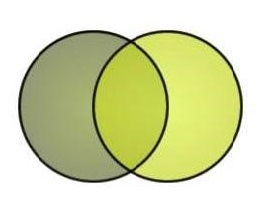# schrodinger equation

n=3

a: Schrodinger equation ~
b: Newton’s Law in classical mechanics

What:

"Schrodinger equation is like Newton's Law in classical mechanics. It predicts the future behavior of quantum mechanical systems by describing the time evolution of the associated wavefunctions. A system's wavefunction gives a complete description its quantum state."

Useful?
Writer: Joel Bombile
LCC:
Where:
Date: Oct 13 2018 12:17 PM

a: Schrodinger equation ~
b: F=ma of quantum mechanics

What:

"&#968; represents the particle. In the Copenhagen interpretation, this is the most complete description of a physical system. It describes how a particles wave equation (also called state function) changes with time and space. To find this, you solve the Schrodinger equation. The Schrodinger equation is like the F=ma of quantum mechanics. For the most part, you will just plug in what you need the equation and solving it will yield &#968;."

Useful?
Writer: Hugger
LCC:
Where:
Date: Oct 13 2018 12:23 PM

Ficks's Law For Wave Diffusion..?

a: Schrodinger equation ~
b: diffusion equation

What:

"In 1941 Richard Feynman pointed out that amplitudes in quantum theory could be worked out by using path integrals that sum with appropriate weights contributions from all possible histories of a system. (The Schrödinger equation is like a diffusion equation in imaginary time, so the path integral for it can be thought of as like an enumeration of random walks. The idea of describing random walks with path integrals was discussed from the early 1900s.)"

Useful?
Writer: Not Stated
LCC:
Where:
Date: Oct 13 2018 12:25 PMMETAMIA is a free database of analogy and metaphor. Anyone can contribute or search. The subject matter can be anything. Science is popular, but poetry is encouraged. The goal is to integrate our fluid muses with the stark literalism of a relational database. Metamia is like a girdle for your muses, a cognitive girdle.